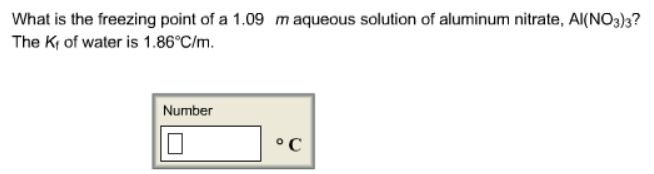Chemistry Practice Problems Freezing Point Depression Practice Problems Solution: What is the freezing point of a 1.09 m aqueous sol...

# Solution: What is the freezing point of a 1.09 m aqueous solution of aluminum nitrate, AI(NO 3)3? The Kf of water is 1.86°C/m.

###### Problem

What is the freezing point of a 1.09 m aqueous solution of aluminum nitrate, AI(NO 3)3? The Kf of water is 1.86°C/m.View Complete Written Solution

Freezing Point Depression

Freezing Point Depression

#### Q. Assuming 100% dissociation, calculate the freezing point and boiling point of 2.96 m Na2SO4(aq).

Solved • Wed May 30 2018 12:36:26 GMT-0400 (EDT)

Freezing Point Depression

#### Q. The freezing point of water H2O is 0.00 degree C at 1 atmosphere. A nonvolatile, nonelectrolyte that dissolves in water is sucrose. How many grams of ...

Solved • Wed Apr 18 2018 16:31:20 GMT-0400 (EDT)

Freezing Point Depression

#### Q. The freezing point of ethanol CH3CH2OH is - 117.30 degree C at 1 atmosphere. A nonvolatile, nonelectrolyte that dissolves in ethanol is chlorophyll. I...

Solved • Wed Apr 18 2018 16:15:04 GMT-0400 (EDT)

Freezing Point Depression

#### Q. Which of the following aqueous solutions has the lowest freezing point? The K f of water is 1.86 kg.°C/mol. Calculate the freezing point ONLY for the ...

Solved • Fri Mar 30 2018 16:01:00 GMT-0400 (EDT)